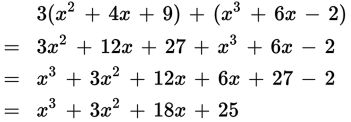# SAT Math Multiple Choice Question 323: Answer and Explanation

### Test Information

Question: 323

8. If A = x2 + 4x + 9 and B = x3 + 6x – 2, what is 3A + B?

• A. 4x2 + 18x + 25
• B. x3 + x2 + 10x + 7
• C. x3 + 3x2 + 18x + 25
• D. 3x3 + 3x2 + 30x + 29

Explanation:

C

Difficulty: Medium

Category: Passport to Advanced Math / Exponents

Strategic Advice: When adding or subtracting polynomial expressions, simply combine like terms (terms that have the same variable part). Pay careful attention to the exponents. To keep things organized, arrange the terms in descending order before you combine them.

Getting to the Answer: Substitute the given expressions for A and B into 3A + B. Distribute the 3 to each term of A and then combine like terms. Be careful-the first term of B is x3, not x2, so these cannot be combined.